If you are a Python developer—whether for data science, big data Spark, or other—you should be using Pandas Python.  This Python package makes data transformation and selecting simple and, many enthusiasts say, fun.

### Python Pandas

When you start studying Pandas you might find that some of the articles on the internet give examples that are overly scientific.  So that would lead you to think this is a package mainly for data scientists, meaning statisticians and mathematicians.  Another reason you might think that is because you often see Pandas examples importing numpy, which is characterized as a “package for scientific computing with Python.” Yet it is true data scientists are thrilled about Pandas. But it is highly useful for everyone else too.  And is it not also true that in the realm of big data, everyone is to some degree a data scientist?

Here we give some examples of how to use Pandas to show how useful it is.  We will show how to:

• Select records using Pandas syntax as you might with SQL
• Write a Pandas dataframe to a Spark dataframe
• Convert a Python dictionary to a Pandas dataframe

### Definitions

But first some definitions:

Dictionary—is a python structure.  We mention it here because we show how to convert that a Python dictionary to a Pandas dataframe.  A dictionary is like a hashtable.  It has keys and values, like this:

{key : value}

Data frame—is the main Pandas data structure.  Think of it like a spreadsheet with rows and columns.

Series—think of this as a dataframe with just one column.

Basic Intro to Data Frames

Here are tersely worded explanations of data frame basics and how to reference data from Pandas.org.  Below we give a more gentle explanation.

To reference data you need to understand Python slice notation. The Panda slice notation expands the NumPy ndarray (n-dimensional array) notation using “[“, ”]”, and “:” .  Plus you can use column names.

Suppose you create a dataframe like shown below. This creates a dataframe with two columns, first named “a” and the second name “b” with 10 rows of data:

pd.DataFrame({‘a’:range(20,30),’b’:[11,12,13,14,15,16,17,18,19,20]})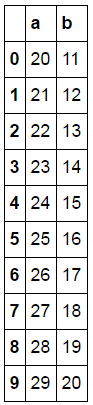Then it can be sliced as follows:

 df[‘a’] Creates series with one column: column a.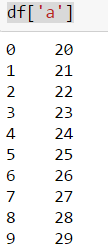df[2:4] Rows 2 to 4: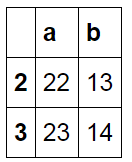df[:4} First 4 rows: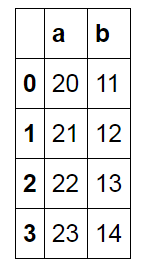df[4:] Start at row 4 and go to end: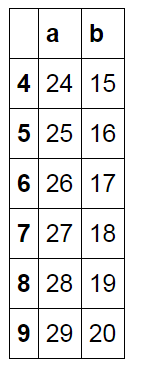### Convert a Dictionary to a Data Frame

Look at this complicated looking nested dictionary below.  It gives weather readings by date. If you wanted to convert this to a Python dataframe or tuple to write to Spark you might have to make some kind of complicated loop.  But with Pandas you can do it with one short command.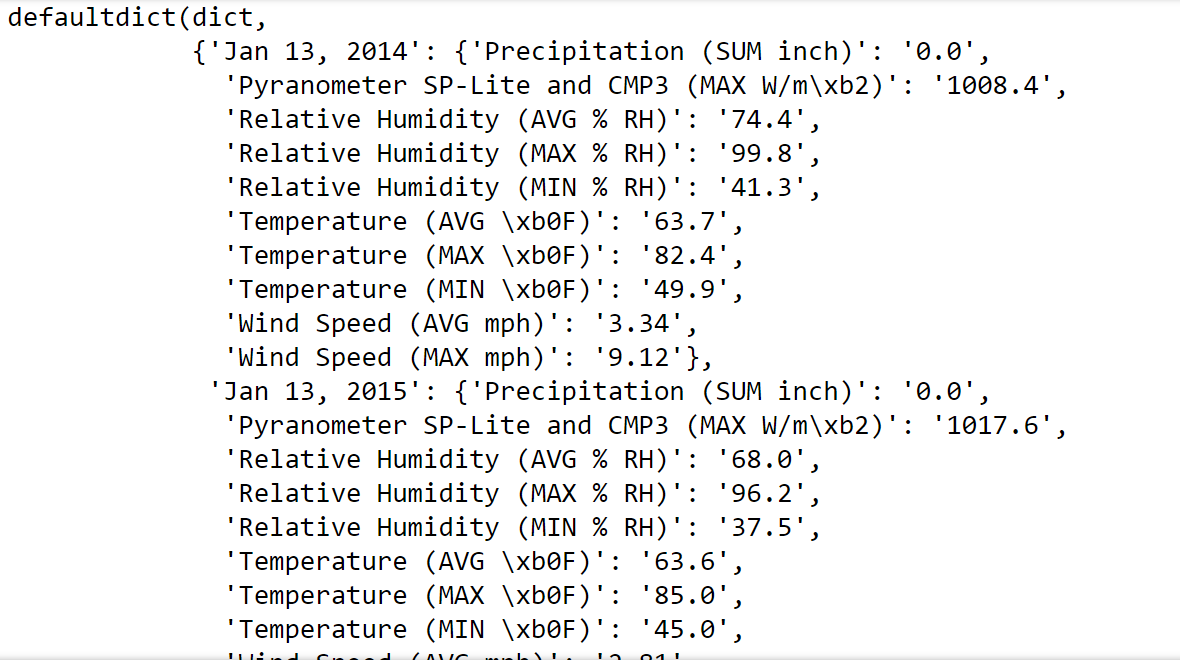import pandas as pd

weatherDF= pd.DataFrame.from_dict(weatherDict, orient= “index” )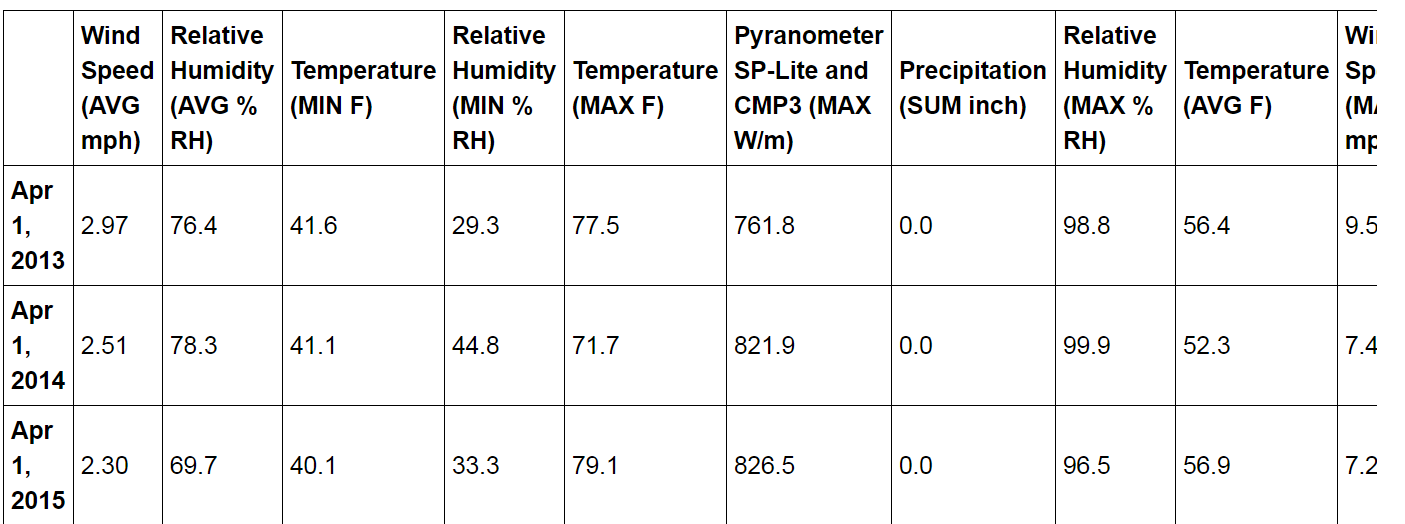Notice that it used the “Date” from the outermost part of the dictionary as the row labels in the data frame.  That is different that the simpler dictionary we made above which simply numbered each row.  Also you could have changed the dataframe to have the dates as the columns and the weather readings at the rows by using:

orient = “columns”

### Select Records as You Might With SQL

How do you do SQL-type operations on Pandas dataframes?  Pandas explains it here.  Below we give our own examples.

First, let’s rename those column names in our sample dataframe to make them simpler so we can do some SQL operations on this.  We use:

weatherDF.rename(columns={‘Wind Speed (MAX mph)’:’maxWind’},inplace=True)

(and so forth)

To produce this:In SQL we might write this to select certain columns based on certain conditions:

select aveTemp, maxTemp, minTemp when aveTemp >= 90

In Pandas we write that like this:

may2014 = weatherDF[[‘aveTemp’, ‘minTemp’, ‘maxTemp’]]

may2014[(may2014[‘maxTemp’] >= 90)]

The first statement creates the dataframe May2014 with three columns and the second pulls just those rows where the maxTemp is >= 90.

[See Also:Introduction to “Java to Objective-C” and When to Use this Translation Tool]

### Write Data to Spark

Here we give an example of how to save Pandas dataframes to Spark.   Spark has recently added that ability so that in addition to creating spark RDDs (resilient distributed datasets) from Python tuples, lists, and dictionaries you can do that from Pandas dataframes. Of course doing that would also let you work with the data we just looked at above using SparkSQL if you prefer to use SQL.

from pyspark import SparkContext

from pyspark.sql import SQLContext

sqlCtx = SQLContext(sc)
sqlCtx.createDataFrame(weatherDF).show()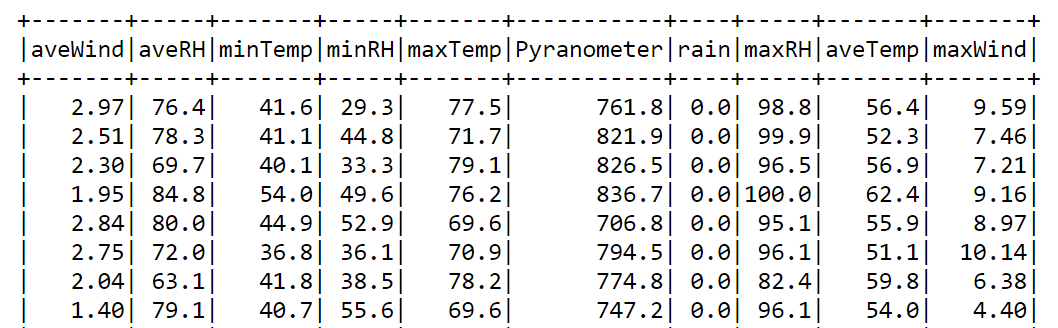Here is some additional reading from DataBricks for some examples of how you might use the Spark abilities of Panda dataframes.

Everything you need to know about outsourcing technology development

Access a special Introduction Package with everything you want to know about outsourcing your technology development. How should you evaluate a partner? What components of your solution that are suitable to be handed off to a partner? These answers and more below.

Introduction Package

Zymr blogger